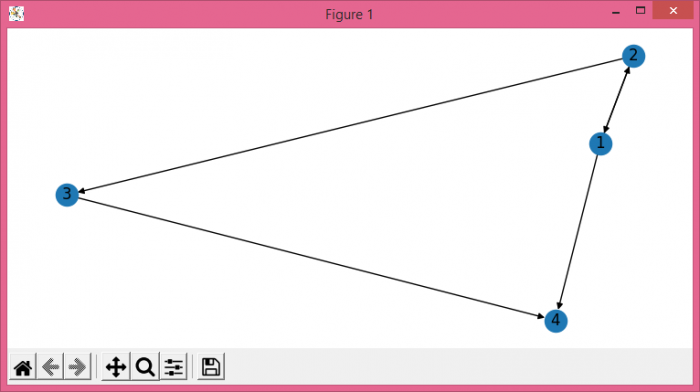# How to show node name in Matplotlib graphs using networkx?

To show node name in graphs using networkx, we can take the following steps −

• Set the figure size and adjust the padding between and around the subplots.
• Initialize a graph with edges, name, or graph attributes.
• Draw the graph G with Matplotlib using draw() method. Set with_labels to True.
• To display the graph, we can use show() method.

## Example

import matplotlib.pylab as plt
import networkx as nx

plt.rcParams["figure.figsize"] = [7.50, 3.50]
plt.rcParams["figure.autolayout"] = True

G = nx.DiGraph()
plt.show()